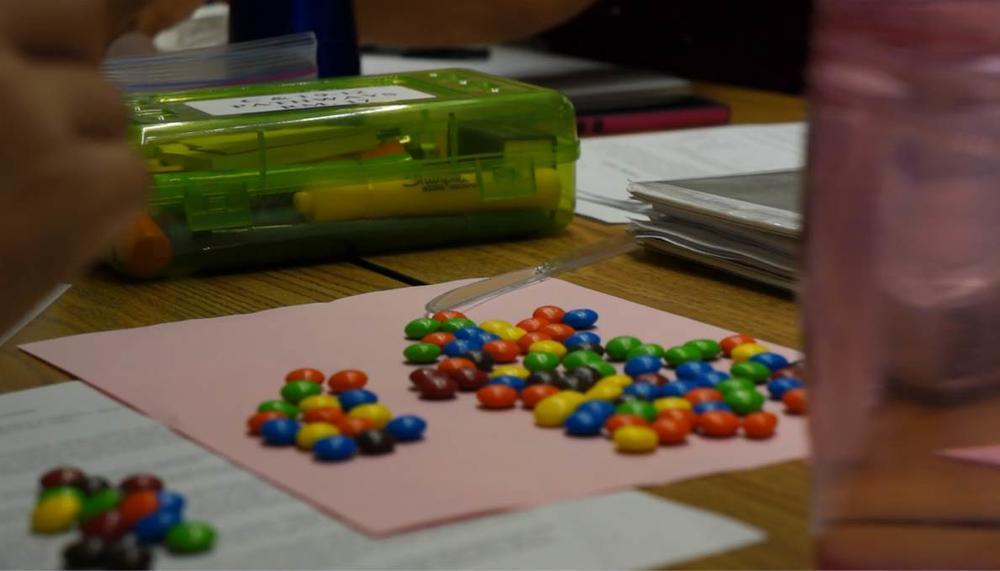MathematicsHOW HAS COMMON CORE MATHEMATICS INSTRUCTION CHANGED THE CLASSROOM?

• Students continually ask themselves, "Does this make sense?"
• Students attend to the meaning of quantities, no just how to compute the them.
• Students listen to the arguments of others, and ask useful questions to determine if an argument makes sense.
• Students apply the mathematics they know to solve everyday problems.
• Students use technological tools to deepen their understanding of mathematics.
• Students communicate precisely with others and try to use clear mathematical language when discussing their reasoning.
• Students apply general mathematical patterns, rules or procedures to specific situations.
• Students see the overall process of the problem and still attend to details in the problem-solving steps.